## How to Use deMon2k

The deMon2k program implements DFT in the Kohn-Sham formulation. It uses the linear combination of Gaussian type orbital (LCGTO) method. In this framework, the Kohn-Sham orbitals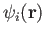are expanded in an atomic orbital basis:(1)

Here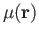denotes an atomic orbital (built from contracted Gaussian basis functions) andthe corresponding molecular orbital coefficient. With this expansion, the electronic density is:(2)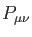is an element of the (closed-shell, also called non-spin-polarized in the DFT literature) density matrix defined as: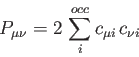(3)

Using the LCGTO expansions for the Kohn-Sham orbitals (1.1) and the electronic density (1.2), the Kohn-Sham self-consistent field (SCF) energy expression  can be expressed as:(4)

The total energy is the sum ofand the nuclear repulsion energy, which can be calculated analytically. In (1.4),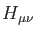are elements of the core Hamiltonian matrix. They are built from the kinetic and nuclear attraction energy operators of the electrons and describe the distribution of an independent electron in the nuclear framework. The second term in (1.4) is the Coulomb repulsion energy of the electrons. In the short-hand notation for the four-center electron repulsion integrals (ERIs) the symbolrepresents the two-electron Coulomb operator and separates functions of electron 1 from those of electron 2. In contrast to Hartree-Fock theory, the calculations of the Coulomb and exchange energies are separate in Kohn-Sham DFT. Calculation of the exchange-correlation energy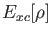requires numerical integration. In deMon2k, thescaling of straight-forward calculation of the Coulomb repulsion energy is avoided by introducing an auxiliary function density . This approximated densityis expanded in primitive Hermite Gaussians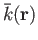which are centered on the atoms [51,52]:(5)

The primitive Hermite Gaussian auxiliary functions are grouped in auxiliary function sets that share the same exponent [53,54]. For this reason, they usually are denoted as s, p, d etc. auxiliary function sets. With the LCGTO expansion forand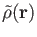we obtain the following approximate SCF energy: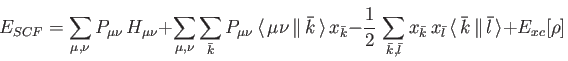(6)

Therefore, only three-center electron repulsion integrals are necessary for the SCF and energy calculation in deMon2k. This represents the density fitting Kohn-Sham method available in deMon2k. It is activated by the keyword VXCTYPE BASIS (see Section 4.2.1 for more details about the VXCTYPE keyword). However, by default (VXCTYPE AUXIS), the approximated density is also used for the calculation of the exchange-correlation energy: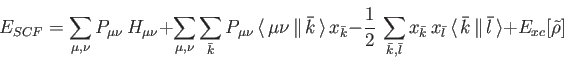(7)

This is the auxiliary density functional theory (ADFT) energy expression. For more details on ADFT, see the reviews [55,56,57,58,59]. Typically, the optimized ADFT structure parameters are indistinguishable from their full DFT counterparts even for weakly bound systems (here the use of the GEN-A2* auxiliary function set is recommended; see Section 4.3.3 and Appendix A). For binding energies, ADFT and Kohn-Sham results typically deviate by less than 1 kcal/mol if GEN-A2* or larger auxiliary function sets are used. Thus, the differences between ADFT and Kohn-Sham DFT geometries and bond energies are usually in the range of the accuracy of the underlying approximate exchange-correlation functional. Because of the considerable savings in computational time, we suggest to use ADFT for all studies including frequency analysis and property calculations. The VXCTYPE BASIS option Eq. (1.6) should be employed only if direct comparison with four-center DFT calculations is required. It should be noted that the default setting for the auxiliary functions is GEN-A2, independent of which energy expression is used (see Section 4.3.3). For all theoretical models available in deMon2k, VXCTYPE AUXIS results can be used as a restart guess (GUESS RESTART; see Section 4.5.5) for VXCTYPE BASIS calculations.

The most frequently encountered problem in DFT calculations is the failure to achieve SCF convergence. Usually this is caused by the small energy gap between the highest occupied (HOMO) and lowest unoccupied (LUMO) molecular orbital. In deMon2k, the DIIS procedure (Section 4.5.8) is activated by default. For a small HOMO-LUMO gap, DIIS may be counterproductive and should be switched off. There are several options available in deMon2k to achieve SCF convergence. Most important are modifications of the choice of the starting GUESS (Section 4.5.5) and the MIXING (Section 4.5.6) of the old and new (auxiliary) densities as well as enlargement of the HOMO-LUMO gap by the level-SHIFT (Section 4.5.7) procedure. If a static level-shift is employed it is advisable to check the orbital energies and occupations at the HOMO-LUMO gap by use of the PRINT keyword (Section 4.12.2). Other relevant keywords to alter or achieve SCF convergence are MOEXCHANGE (Section 4.4.3), FIXMOS (Section 4.4.5) and SMEAR (Section 4.4.6). For atomic calculations, the CONFIGURE keyword (Section 4.4.7) should be used in order to ensure SCF convergence.

In deMon2k 5.0 the calculation of Hartree-Fock energies by the variational fitting of the Coulomb and Fock potentials is also available. The corresponding SCF energy has the form :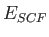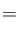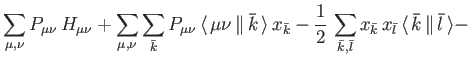(8)

Note that the same auxiliary function sets are used for the Coulomb and Fock potential fitting. As a result, the approximated Hartree-Fock energy, Eq. (1.8), is self-interaction free. To obtain a computationally efficient methodology the Fock potential fitting is performed with localized molecular orbitals . This yields a computationally efficient and very accurate approximate Hartree-Fock energy expression that only requires three-center ERIs. Deviations with respect to four center ERIs total energies are below 1 kcal/mol if GEN-A2* auxiliary function sets are used. With this development hybrid functionals such as B3LYP [62,63], PBE0 [64,65] and M06-2X  are now available in deMon2k .

For QM/MM calculations in deMon2k 5.0 the following energy expression is used :(9)

The QM energy,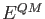, can be calculated with any of the above discussed SCF energy expressions given in Eqs. (1.6) to (1.8) or corresponding hybrid functional expressions. In all cases the core Hamiltonian matrix elements,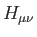, are augmented in order to take into account the electrostatic embedding of the QM system by the MM region:(10)

In Eq. (1.10)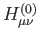denotes original core Hamilton matrix elements of the QM system and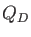denotes the atomic charges of the MM atoms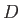. The general form of the nuclear attraction type operator.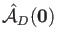, is given by:(11)

This general definition permits immediately the inclusion of MM atoms with higher point moments. Note that Eq. (1.10) is also used for pure electrostatic embedding  with the EMBED keyword (see 4.2.6). In both cases asymptotic expansions for the long-range nuclear attraction type integrals are implemented in order to improve computational efficiency . Another part of the QM energy in Eq. (1.9) is the MM augmented nuclear repulsion energy,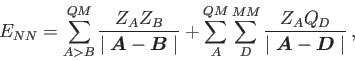which can be calculated analytically from the structure of the QM/MM system. Because the so-defined QM energy contains all quantum mechanical terms plus the electrostatic embedding from the MM region the Kohn-Sham or Hartree-Fock matrix elements can be defined as partial derivatives of this energy with respect to density matrix elements.

The second term in Eq. (1.9) contains the mechanical interaction energy between the QM and MM regions. It is expressed in the form of a Lennard-Jones potential: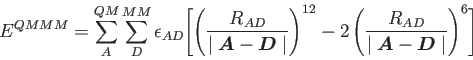(12)

The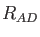are combinations of the van der Waals radii of QM atom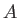and MM atom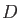. By default these radii are taken from the MM force field. The parameterdefines the depth of the Lennard-Jones potential. As for the van der Waals radii it is also taken from the MM force field. Therefore, an MM atom type has to be assigned to each QM atom in the input. This is done with the QM/MM keyword (Section 4.2.4).

The last term in Eq. (1.9) is the MM energy. In deMon2k 5.0 it can contain the following terms:(13)

The first four terms in Eq. (1.14) denote bond stretching, angle bending, dihedral torsion and Urey-Bradley energy terms. Their calculation requires molecular connectivity information that is usually given in the input along with the geometrical definition of the MM atoms under the GEOMETRY keyword (see 4.1.1). As an alternative, the automatic generation of molecular connectivity information on the basis of the distances between MM atoms is also available. The last two terms in Eq. (1.14) represent van der Waals and point-charge interaction energies between the MM atoms. The force fields for MM and QM/MM calculations available in deMon2k are OPLS-AA  and AMBER . They are selected by the FORCEFIELD keyword (see 4.2.3) and read from the FFDS (force field dataset) file. For these MM and QM/MM calculations all deMon2k functionalities, such as geometry optimization, transition state finding, molecular dynamics, frequency analysis etc., are available. Also property calculations for the QM system in QM/MM calculations are possible .

Besides the internal MM capability, deMon2k can also be externally interfaced with force fields. To this end a standard interface output for CHARMM  can be activated with the QM/MM keyword [44,46,73].

By default, the ERIs are calculated in each SCF cycle (direct SCF) using recurrence relations for near-field ERIs [20,51] and double asymptotic expansions  for far-field ERIs. This approach minimizes the random access memory (RAM) demand of deMon2k. If sufficient RAM is available the code performance can be improved by the MIXED option of the ERIS keyword (see 4.5.4). The RAM usage of deMon2k can be monitored by PRINT RAM (see Section 4.12.2 for more details). It also should be noted that, for larger systems, the linear algebra steps in deMon2k may become a bottleneck. With the keywords MATDIA and MATINV (see 4.11.2 and 4.11.3) alternative diagonalizers and matrix inversion techniques can be selected.

Several optimization and transition state search algorithms are implemented in deMon2k. For structure optimization, the default setting is the Levenberg-Marquardt restricted step method in delocalized internal redundant coordinates. This method has excellent convergence behavior and is very robust. However, it requires an iterative back transformation of the coordinates. Thus, to reach tight structure convergence, it may be necessary to switch to Cartesian coordinates at the end of the optimization (see 4.6.1). For ultimate accuracy, this might be combined with a Hessian calculation in each optimization step (UPDATE EXACT; Section 4.6.5). If effective core potentials (ECPs), Section 4.3.4, or model core potentials (MCPs), Section 4.3.5, are used, care must be taken regarding the accuracy of the gradients. Here it may be necessary to tighten the numerical integration threshold with the GRID keyword (see 4.3.6). Usually a FINE grid will be sufficient. The same holds for weak and nonbonded interactions. For the local transition state search, we recommend starting the optimization from a calculated Hessian (see 4.6.5) or restarting it from a frequency analysis (the Hessian from the frequency analysis is then used in the first optimization step). If a SADDLE point interpolation (Section 4.6.2) is to be performed, the starting points must be local minima, i.e. reactants and products. All optimizations and interpolations can be restarted with the deMon.new and deMon.mem files. These must be copied into the new input file deMon.inp and the corresponding restart file deMon.rst. The new input file may be modified and extended but the molecular geometry definitions must be left untouched in order to guarantee a successful restart run.

Born-Oppenheimer molecular dynamics (BOMD) simulations are initialized by the DYNAMICS keyword (see 4.7.1). In these calculations a trajectory file deMon.trj is created which can be large! For compatibility reasons, the trajectory file is written in ASCII (Note that *********************** are used as separations in this file). It should not be modified. The data from the trajectory file can be used to restart BOMD runs or to analyze them (Sections 4.7.2 and 4.7.3). Because BOMD runs may take weeks, we recommend that regular snapshots of the deMon working directory be produced from which restarts are possible. During such a copy the trajectory, deMon.trj, and new input file, deMon.new, must be unchanged. With these files, a restart run is possible just as in the case of structure optimizations, i.e. the deMon.new must be copied into the new input file deMon.inp. If requested, the restart file can also be used, e.g. for a restart density (GUESS RESTART; see 4.5.5). However, this is not mandatory.

Usually the default settings of deMon2k are sufficient for standard calculations. However, if extended basis sets are used or higher accuracy is required, it may be necessary to adjust the accuracy and performance settings of the code. This is achieved by the keywords GRID, SCFTYPE and ERIS (see 4.3.6, 4.5.1 and 4.5.4) for the electronic structure calculation, the keyword OPTIMIZATION (see 4.6.1) for the structure optimization and the keywords MATDIA and MATINV (see 4.11.2 and 4.11.3) for the linear algebra parts of the code. The keywords WEIGHTING, QUADRATURE and CFPINTEGRATION control the accuracy settings for the numerical integration (see 4.11.5, 4.11.6 and 4.11.7). The keyword DAVIDSON (4.11.4) controls the iterative diagonalization in time-dependent DFT calculations. In general, modification of the standard settings may alter the performance and accuracy of the code quite substantially. Therefore, such modifications should be tested carefully before being used for production runs.